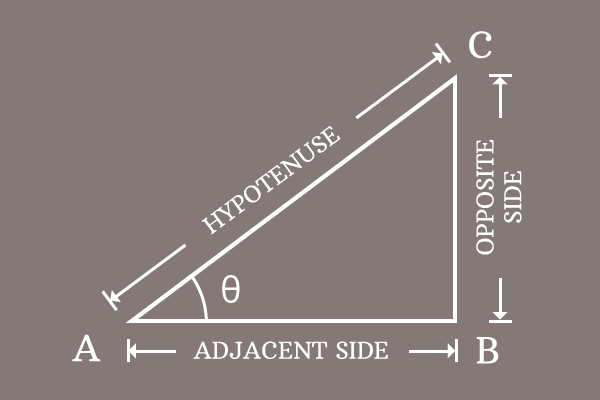Pythagorean Identity of Secant and Tangent functions

The subtraction of square of tangent function from secant function at an angle equals to one, is called Pythagorean identity for secant and tangent functions.

Formula

$\sec^2{\theta} \,-\, \tan^2{\theta} = 1$

Proof$\Delta CAB$ is a right angled triangle, whose opposite side, adjacent side and hypotenuse are denoted by $\overline{BC}$, $\overline{AB}$ and $\overline{AC}$ respectively. The lengths of the associated sides are $BC$, $AB$ and $AC$ respectively.

Expressing Relation between sides

The relation between the sides of the right angled triangle can be written in mathematical form according to the Pythagorean Theorem.

${AC}^2 = {BC}^2 + {AB}^2$

$\implies {BC}^2 + {AB}^2 = {AC}^2$

Try to express the mathematical equation in terms of trigonometric functions. It is possible to express it in terms of secant and tangent functions if the equation is divided by the square of the length of the side $AB$.

$\implies \dfrac{{BC}^2 + {AB}^2}{{AB}^2} = \dfrac{{AC}^2}{{AB}^2}$

$\implies \dfrac{{BC}^2}{{AB}^2} + \dfrac{{AB}^2}{{AB}^2} = \dfrac{{AC}^2}{{AB}^2}$

$\implies \Bigg(\dfrac{BC}{AB}\Bigg)^2 + \Bigg(\dfrac{AB}{AB}\Bigg)^2 = \Bigg(\dfrac{AC}{AB}\Bigg)^2$

$\implies \Bigg(\dfrac{BC}{AB}\Bigg)^2 + 1 = \Bigg(\dfrac{AC}{AB}\Bigg)^2$

Expressing the Equation in terms of Secant and Tangent

Now, write the ratios of the sides in terms of secant and tangent functions.

$\dfrac{BC}{AB} = \tan{\theta}$

$\dfrac{AC}{AB} = \sec{\theta}$

Replace the ratios of the sides by the respective trigonometric functions to transform the equation in the form of trigonometric functions.

$\implies (\tan{\theta})^2 + 1 = (\sec \theta)^2$

$\implies \tan^2{\theta} + 1 = \sec^2{\theta}$

$\implies 1 = \sec^2{\theta} \,-\, \tan^2{\theta}$

$\,\,\, \therefore \,\,\,\,\,\, \sec^2{\theta} \,-\, \tan^2{\theta} = 1$

Therefore, it is proved that the subtraction of square of tangent function from square of secant function at an angle is equal to one. The trigonometric identity is actually derived as per Pythagoras Theorem. Thus, it is known as the Pythagorean identity for secant and tangent functions.

Latest Math Problems
Email subscription
Math Doubts is a best place to learn mathematics and from basics to advanced scientific level for students, teachers and researchers. Know more